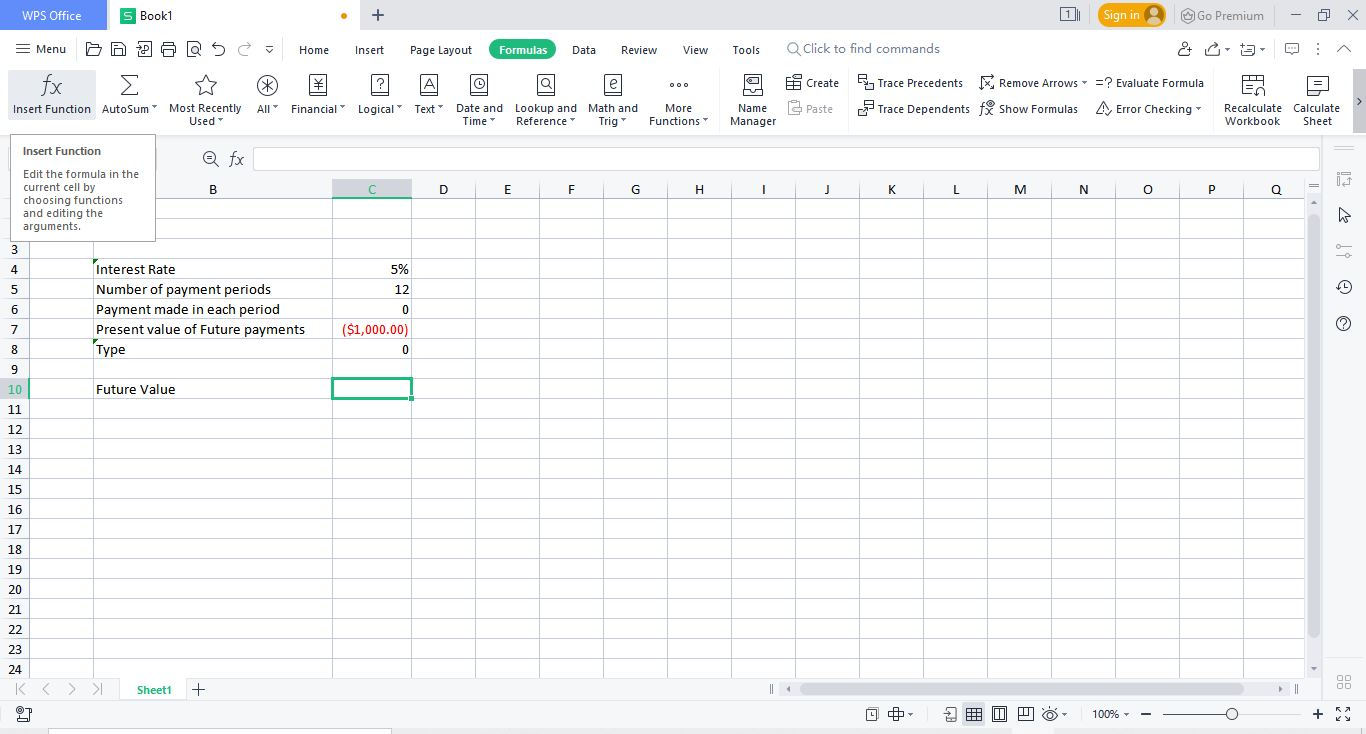# How to Use Future Value Function in Excel

August 9, 2022
2.3K Views
0

A free Office suite fully compatible with Microsoft OfficeExcel can be used for different sorts of calculations and statistical analysis as well. Sometimes while doing statistical analysis you require to calculate future value. Manual calculations of determining the future value are complex and laborious. However excel provides a simple solution to this problem i.e. formula of Future value excel WPS (2016/2019/mac/online). Using this simple formula you can calculate future values very easily in your statistical analysis.

This article covers the basic methods to use future value formula in excel (2016/2019/mac/online). Once you go through this article you will be able to easily use future value formula in excel. Let’s cover the simple methodologies of using future value formula in excel WPS/ (2016/2019/mac/online)

Three different ways of calculating future value in excel.

Using Insert function button in excel to calculate future value

You can calculate future value in excel by using insert function button by following simple below mentioned steps and picture illustrations.

Steps:

1.Open WPS Excel /Spreadsheet file where you want to calculate future value in excel.

2.Click on the cell where you want to calculate future value.

3.Go to Formulas Tab.There will be an Insert formula button in it.4.Click on this button.A new dialogue window shall appear.In the search for a formula bar write “FV”.FV (Future Value) shall appear in the below list.

5.Click on FV and press OK.A new function argument window shall appear.

• To calculate Future value, five different parameters are required.

•  i.e.Rate (Interest rate)

• Nper (Number of Payment periods)

• Pmt (Payment made in each period, It must be in negative)

• Pv (Present value of future payments, It is optional. If it is omitted then Excel takes it as zero)

• Type (When Payments are due. It is optional as well, 1 = beginning of period and 0 = end of period. By default it is taken as 0 by excel)

You can either enter the values of these 05 parameters or you can use excel value links as used in picture illustrations in this article.

After entering all five parameters, press enter.Future value is calculated.

Using Financial formula section in excel to calculate future value in excel

You can calculate future value by using financial formula section by following simple below mentioned steps and picture illustrations.

Steps:

1.Open WPS Excel /Spreadsheet file where you want to calculate future value in excel.

2.Click on the cell where you want to calculate future value.

3.Go to Formulas Tab.There will be an financial formula button in it.

4.Click on this button.A drop down list shall appear.In the list, find FV and click on it

5.A new function argument window shall appear.

To calculate Future value, five different parameters are required.

•  i.e.Rate (Interest rate)

• Nper (Number of Payment periods)

• Pmt (Payment made in each period, It must be in negative)

• Pv (Present value of future payments, It is optional. If it is omitted then Excel takes it as zero)

• Type (When Payments are due. It is optional as well, 1 = beginning of period and 0 = end of period. By default it is taken as 0 by excel)

You can either enter the values of these 05 parameters or you can use excel value links as used in picture illustrations in this article.

After entering all five parameters, press enter.Future value is calculated.

Manually entering Future value Formula in excel to calculate future value

You can calculate future value by manually entering future value formula by following simple below mentioned steps and picture illustrations.

Steps:

1.Open WPS Excel /Spreadsheet file where you want to calculate future value in excel.

2.Click on the cell where you want to calculate future value.Write “=FV”, the press Tab.

Future value formula is initiated.

• To calculate Future value, five different parameters are required.

•  i.e.Rate (Interest rate)

• Nper (Number of Payment periods)

• Pmt (Payment made in each period, It must be in negative)

• Pv (Present value of future payments, It is optional. If it is omitted then Excel takes it as zero)

• Type (When Payments are due. It is optional as well, 1 = beginning of period and 0 = end of period. By default it is taken as 0 by excel)

You can either enter the values of these 05 parameters or you can use excel value links as used in picture illustrations in this article.

After entering all five parameters, press enter.Future value is calculated.

In this way you can easily calculate Future value in excel in the WPS excel/spreadsheet.

This article has covered the simplest ways on calculating Future value in WPS Excel/Spreadsheet. You can use these simple steps as explained above and easily calculate Future value in excel WPS Excel/Spreadsheet whenever required.

Hopefully you have learned How to use calculate Future value excel in WPS Excel/Spreadsheet?  If you want to know more about Excel features, you can follow WPS Academy to learn.